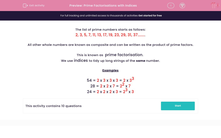# Understand Prime Factorisations with Indices

In this worksheet, students will select the correct prime factorisation for the given numbers.This content is premium and exclusive to EdPlace subscribers.Key stage:  KS 2

Curriculum topic:   Maths and Numerical Reasoning

Curriculum subtopic:   Factors and Multiples

Difficulty level:#### Worksheet Overview

The list of prime numbers starts as follows:

2, 3, 5, 7, 11, 13, 17, 19, 23, 29, 31, 37........

All other whole numbers are known as composite and can be written as the product of prime factors.

This is known as prime factorisation.

We use indices to tidy up long strings of the same number.

Examples

54 = 2 x 3 x 3 x 3 = 2 x 33

Test it out if you're not sure how we got that!  3 x 3 x 3 = 27 x 2 = 54  - does that make sense now?

We've got one 2 and three lots of 3. We write that as 2 x 33

Let's look at another couple of examples!

28 = 2 x 2 x 7 = 22 x 7

24 = 2 x 2 x 2 x 3 = 23 x 3